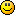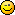# Help my Maths. What are the other "illions"? Millions, Billions, Trillions, the

1. Help my Maths. What are the other "illions"?  Millions, Billions, Trillions, then What?

2. After trillion comes quadrillion, quintillion, sextillion, septillion, octillion, nonillion, decillion, undecillion, duodecillion, quattuordecillion, quindecillion, sexdecillion, septendecillion, octodecillion, novemdecillion, vigintillion and centillion

3. Lol Nancy. This tickled me, but then I read it again and thought you could be right. Didn't know there were so many of them. Thanks for answering.4. Who needs all the extra forms of 'illions' when you can go all the way with the term 'googolplex'...
A googolplex is "ten raised to the power of one googol"...trust me, there is a lot of zeros involved here.
Happy formulating...   Thankfully, I haven't had to use this type of complex math, as my current lifestyle doesn't call for it.5. Lady E - Take the easy way out. Instead of remembering all those different "illions", just do as I do and use the word, gazillion.
It works for me.

6. We all probably need to learn to go beyond trillion...The national debt will be there before we all know it!!   :-)

7. Gazillionfillionzillion....I think thats it

8. Reminds me of a joke:

Don Rumsfeld walks into the Oval office to report on the war on terrorism. As part of the report, he mentions that three Brazilian soldiers were killed in Iraq. W replies,
"Oh, that's terrible! How'm I gonna 'splain that to th' 'Merican people!? How're we gonna replace 'em? Are we gonna hafta reinstate th' draft?.....An' exactly how many is a Brazilian, anyway?"

9. Should I add new -illions- like quadrillion (1,000,000,000,000,000) or five sets of 3 zeros, next will be quintellion (if I'm not mistaken). Mostly, Math terms have Greek word affixes or prefixes (because of Phytagoras and other ancient mathematicians from Greece or Europe).  Please follow this link:

http://gwydir.demon.co.uk/jo/numbers/greek/index.htm

10. According to US norms

10^6=million
10^9=billion
10^12=trillion
10^18=quintillion
10^21=sextillion
10^24=septillion
10^27=octillion
10^30=nonillion
10^33=decillion
10^36=undecillion
10^39=duodecillion
10^42=tredecillion
10^45=quattordecillion
10^48=quindecillion
10^51=sexdecillion
10^54=septemdecillion
10^57=octodecillion
10^60=novemdecillion
10^63=vigintillion